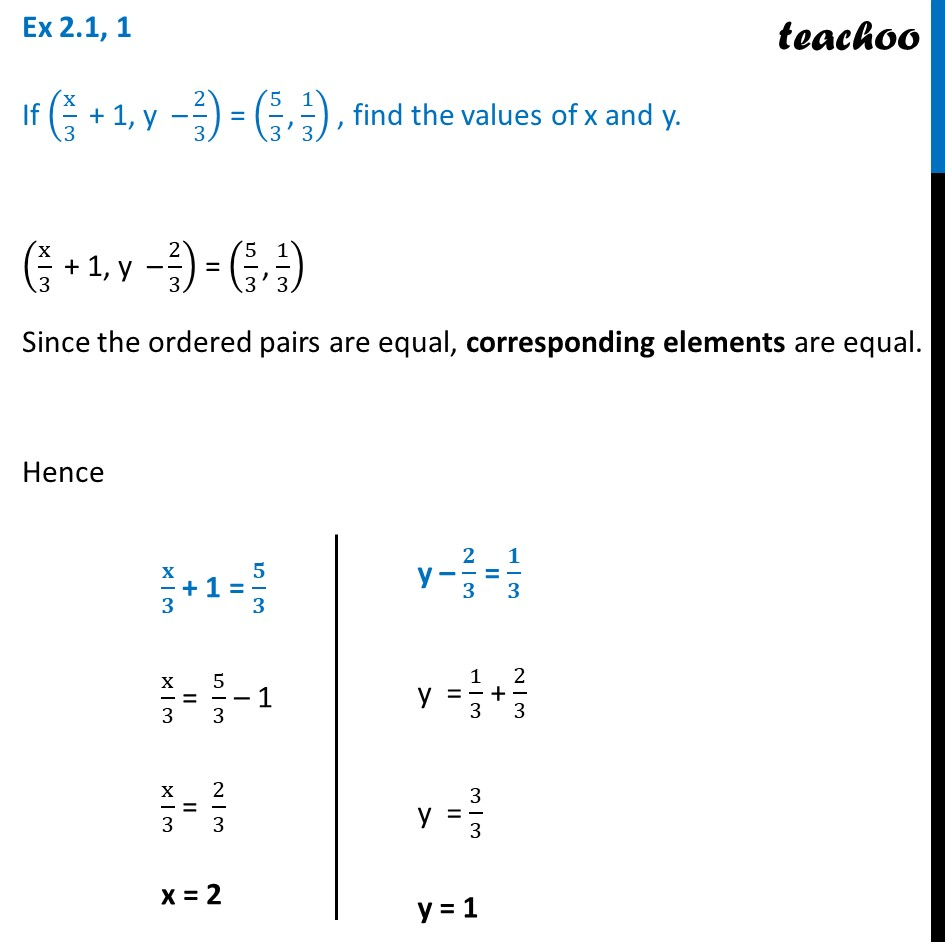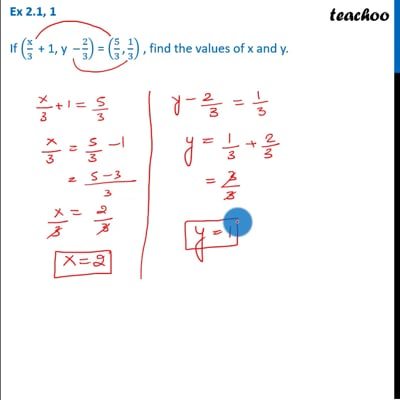Ex 2.1

Chapter 2 Class 11 Relations and Functions
Serial order wiseThis video is only available for Teachoo black users

Solve all your doubts with Teachoo Black (new monthly pack available now!)

### Transcript

Ex 2.1, 1 If (x/3 " + 1, y –" 2/3) = (5/3 "," 1/3) , find the values of x and y. (x/3 " + 1, y –" 2/3) = (5/3 "," 1/3) Since the ordered pairs are equal, corresponding elements are equal. Hence 𝐱/𝟑 + 1 = 𝟓/𝟑 x/3 = 5/3 – 1 x/3 = 2/3 x = 2 y – 𝟐/𝟑 = 𝟏/𝟑 y = 1/3 + 2/3 y = 3/3 y = 1